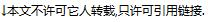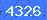# 电阻变化型温度传感器的输入转换电路R(T)=R(0)+aT

1.分压法：2.电阻电桥法：R(T)=R(0)+αTR+R(0)>>aT时，则感测电路的输出电压可简化为3.定电流负载接地法V(T)=I ref ×R(T)=I ref ×(R(0)＋αT)

4.定电流负载浮接法V(T)＝I ref ×R(T)
＝I ref ×(R(0)＋αT)

5.有源电桥法发表评论

 验证码:看不清楚,点击刷新